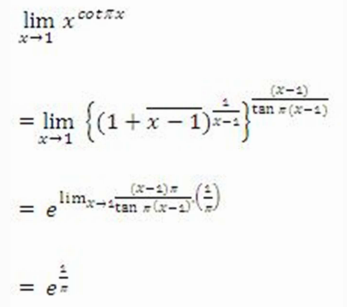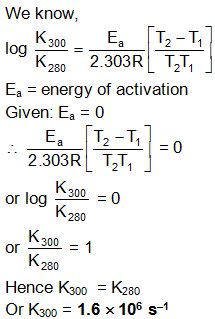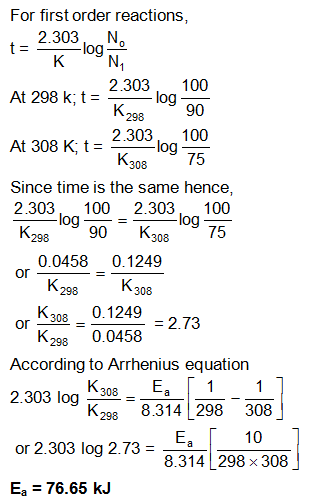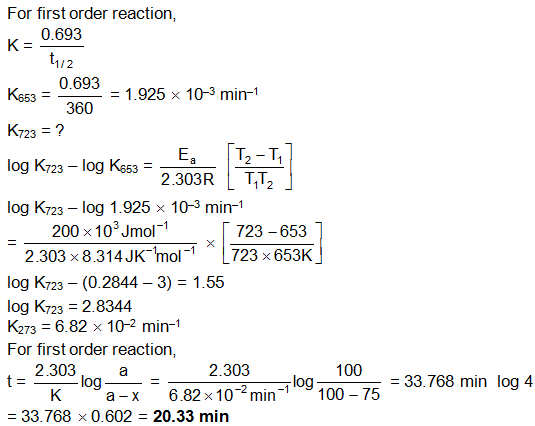Click to Chat

1800-1023-196

+91-120-4616500

CART 0

• 0

MY CART (5)

Use Coupon: CART20 and get 20% off on all online Study Material

ITEM
DETAILS
MRP
DISCOUNT
FINAL PRICE
Total Price: Rs.

There are no items in this cart.
Continue ShoppingSolved Examples

Example : 1

The experimental data for the reaction 2A + B2 → 2AB  is as follows:

Expt. No.

[A]
(mol L-1)

[B2]
(mol L-1)

Rate
(mol L-1 s-1)

1

0.50

0.50

1.6×10-4

2

0.50

1.00

3.2×10-4

3

1.00

1.00

3.2×10-4Write the most probable equation for the rate of reaction giving reason for your answer.

Solution :

From an examination of above data, it is clear that when the concentration of  B2 is doubled, the rate is doubled. Hence the order of reaction with respect to B2 is one.
Further when concentration of A is doubled, the rate remain unaltered. So, order of reaction  with respect to A is zero.
The probable rate law for the reaction will be -dx/dt = k[B2][A]0  = k[B2]
Alternatively Rate = k[B2]α
1.6 × 10-4 = k[0.5]α
3.2 × 10-4 = kα
On dividing we get α = 1
.·. Rate = k[A]0 [B2]   = k[B2]

__________________________________________________________________________

Example : 2

For the reaction   A + 2B → 2C  the following data were obtained.

Expt. No.

Initial concentration
(mol L-1)

Initial reaction rates
(mol L-1min-1)

[A]

[B]

1

1.0

1.0

0.15

2

2.0

1.0

0.30

3

3.0

1.0

0.45

4

1.0

2.0

0.15

5

1.0

3.0

0.15

Write down the rate law for the reaction.

Solution :

Let the rate law be
-dx/dt = k[A]x  [B]y
By keeping the concentration of B constant in experiments (1), (2) and (3) and increasing  concentration uniformly, the rate also increases uniformly, thus,
Rate ∞ [A], i.e., x = 1
By keeping the concentration of A constant in experiments (1), (4) and (5) and increasing  the concentration of B, the rate remains the same.
Hence, y = 0
The rate law is -dx/dt = k[A]

Alternatively method:
From Expt. (1), k[1.0]x [1.0]y  = 0.15 ....(i)
From Expt. (2), k[2.0]x [1.0]y = 0.30 ... (ii)
Dividing Eq. (ii) by Eq. (i),  [2.0]x /[1.0]x  = 0.30/0.15 = 2

So , x = 1

From Expt. (1), k[1.0]x [1.0]y = 0.15 ....(i)
From Expt. (4), k[1.0]x[2.0]y  = 0.15 .... (iii)
Dividing Eq. (iii) by Eq. (i),
[2.0]y /[1.0]y = 1
So , y = 0
Hence, the rate law is -dx/dt = k[A].

___________________________________________________________________________________

Example : 3

For the reaction:  2NO + Cl2 → 2NOCl  at 300 K following data are obtained.

Expt. No.

Initial concentration

Initial rates

[NO]

[Cl2]

1

0.010

0.010

1.2×10-4

2

0.010

0.020

2.4×10-4

3

0.020

0.020

9.6×10-4

Write rate of law for the reaction. What is the order of the reaction?  Also calculate the specific rate constant. .

Solution

Let the rate law for the reaction be

Rate = k[NO]x [Cl2]y

From Expt. (1),

1.2×10-4 = k[0.010]x [0.010]y ... (i)

From Expt. (2),

2.4×10-4 = k[0.010]x[0.020]y  ... (ii)

Dividing Eq. (ii) by Eq. (i),

(9.6×10-4)/(2.4×10-4) = ([0.020]x  )/[0.010]x
or 2 = (2)y
y = 1
From Expt. (2), 2.4×10-4 = k[0.010]x [0.020]y .... (ii)
From Expt. (3), 9.6×10-4 = k[0.020]x [0.020]y .... (ii)
Dividing Eq. (ii) by Eq. (ii),
(9.6 × 10-4)/(2.4 × 10-4) = ([0.020]x )/[0.010]x
or 4 = 2x
x = 2
Order of reaction = x + y = 2 + 1 = 3
Rate law for the reaction is
Rate = k[NO]2 [Cl2]
Considering Eq. (i) again,
1.2 × 10-4 = k[0.010]2 [0.010]
k = (1.2×10-4)/[0.010]3  = 1.2×102 mol-2 litre2 sec-1
________________________________________________________________________________________________________

Example :4

For the hypothetical reaction   2A + B → products  the following data are obtained.

Expt. No.

Initial concentration
(mol L-1)

Initial reaction rates
(mol L-1min-1)

[A]

[B]

1

0.10

0.20

3×102

2

0.30

0.40

3.6×103

3

0.30

0.80

1.44×104

4

0.10

0.40

5

0.20

0.60

6
0.30
1.20

Find out how the rate of the ration depends upon the concentration of A and B and fill in the
blanks.

Solution :

From Expt. (2) and (3), it is clear that when concentration of A is kept  constant and that of B is doubled, the rate increases four times. This shows that the  reaction is of second order with respect to B.
Similarly, from Expt. (1) and (2), it is observed that when concentration of A is increased three times and that of B two times, the rate becomes twelve times. Hence, the reaction is
first order with respect to A.
Thus the rate law for the reaction is
Rate = k[A][B]2

(iv)(v)From Expt. (1), 1.75×10-4 = k[0.0017]x [0.0017]y [1.0]z
From Expt. (4), 3.50×10-4 = k[0.0017]x [0.0017]y [0.5]z
(1.75 × 10-4)/(3.50 × 10-4) = [1.0]z /[0.5]z
or 1/2 = 2z
or 2-1 = 2z
z = -1, i.e., order w.r.to OH-  is -1.
Rate law = k[OCI- ][I- ]/[OH-]
From Expt.(1)k = (1.75×10-4[OH- ])/[OCI-][I-] = (1.75 × 10-4×1.0)/(0.0017×0.0017)  = 60.55 s-1
____________________________________________________________________________

Example : 5

The rate law for the reaction,   2Cl2O → 2Cl2 + O2 at 200oC is found to be : rate = k[Cl2O]2
(a) How would the rate change if [Cl2O] is reduced to one-third of its original value?
(b) How should the [Cl2O] be changed in order to double the rate?
(c) How would the rate change if [Cl2O] is raised to threefold of its original value?

Solution :

(a) Rate equation for the reaction,
r = k[Cl2O]2
Let the new rate be r'; so
r' = k[(Cl2 O)/3]2  = 1/9 r
(b) In order to have the rate = 2r, let the concentration of Cl2O be x.
So 2r = kx2  .... (i)
We know that r = k[Cl2O]2  .... (ii)
Dividing Eq. (i) by (ii),
2r/r = (kx2 )/(k[Cl2O]2 )
or 2 = x2/[Cl2O]2
or x2  = 2[Cl2O]2
or x = √√2 [Cl2 O]
(d) New rate = k[3Cl2O]2  = 9k[Cl2O]2 = 9r
_____________________________________________________________________________________________________

Example : 6

For a reaction in which A and B from C, the following data were obtained from  three experiments:

Expt. No.

Initial concentration
(mol L-1 )

Initial reaction rates
(mol L-1s-1)

[A]

[B]

1

0.03

0.03

0.3×10-4

2

0.06

0.06

1.2×10-4

3

0.06

0.09

2.7×10-4

What is the rate equation of the reaction and what is the value of rate constant?

Solution:

Let the rate equation be k[A]x [B]y
From Expt. (1), 0.3×10-4 = k[0.03]x[0.03]y ... (i)
From Expt. (2), 1.2×10-4 = k[0.06]x [0.06]y ... (ii)
(1.2 × 10-4)/(0.3×10-4) = ([0.06]x [0.06]y)/([0.03]x [0.03]y)  = 2x × 2y = 4 ... (iii)
Similarly from Expt. (1) and (3),
2x ×3y = 9 ....(iv)
Solving Eq. (iii) and (iv),
x = 0, y = 2
Rate equation, Rate = k[B]2
Considering Eq. (i) again,
k = (0.3×10-4)/[0.03]2 = 3.33 × 10-2 mol L-1 s-1
________________________________________________________________________________

Example : 7

For a first order reaction when log k was plotted against , a straight 1/T line with a slope of -6000 was obtained. Calculate the activation energy of the reaction

Solution

Slope = -Ea/2.303R = –6000

Ea = 6000  2.303 8.314

= 1.148  105J

= 27483.935Cal  = 27.48 Kcal

Example : 8

For a reaction, the energy of activation is zero. What is the value of rate constant at 300 K if k = 1.6  106 s–1 at 280 K? (R = 8.31 J K–1 mol–1)

Solution:

We know,___________________________________________________________________________________________

Example : 9

The time required for 10% completion of first order reaction at 298 K is equal to that required for its 25% completion at 308K. If the preexponential factor for the reaction is 3.56 ´ 109 s–1, calculate the energy of activation

Solution:_________________________________________________________________________________

Question : 10

At 380°C, the half-life period for the first order decomposition of H2O2 is 360 min. the energy of activation of the reaction is 200 kJ mol–1, Calculate the time required for 75% decomposition at 450°C.

Solution:Download IIT JEE Solved Examples for Molecularity and Order of Reaction

Related Resources:-

Look here for Syllabus of IIT JEE

You can also refer to  Collision Theory of Reaction Rate

To read more, Buy study materials of Chemical Kinetics comprising study notes, revision notes, video lectures, previous year solved questions etc. Also browse for more study materials on Chemistry here.### Course Features

• 731 Video Lectures
• Revision Notes
• Previous Year Papers
• Mind Map
• Study Planner
• NCERT Solutions
• Discussion Forum
• Test paper with Video Solution